# besace

## quartic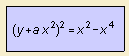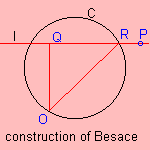The besace is a Lissajous curve. In 1750 Cramer gave the curve its name 1).

The curve can be constructed as follows: given a circle C and a point O draw horizontal lines l which cut the circle in R. Q is the projection of O on l. Then the Besace is the set of points P for which PQ = OR.

The curve is the result of the projection of the pancake curve on a plane through the central z-axis of the curve.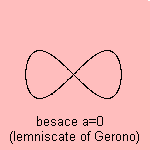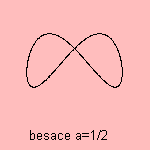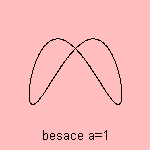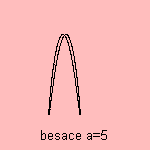For large a, the curve approximates the parabola.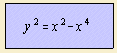The form of the curve is that of ribbon
2), as the other lemniscate, the more famous lemniscate of Bernoulli. Because of the resemblance with the digit 8, the curve is also called the (figure) eight curve.
But I think the figure eight curve that is formed by the hippopede makes a better eight.

Its Cartesian equation resembles the equation of the kampyle of Eudoxus.

The curve was studied by Camille-Christophe Gerono.

notes

1) In German: Quersackkurve.

2) lemniskos (Gr.) = ribbon